# INFERRING BINDING ATTRIBUTES

By inferring cubes, we mean the maximum number of cubes which can be inferred from a given binding attribute. These types of cubes are not uncommon, and they indicate essentially the set of possible answers to a class of queries which can be formulated on the basic binding attributes. This fact highlights the usability of binding attributes.

In database community literature, usability is defined as the ratio of the maximum number of answerable queries to the total number of possible queries, which means any query that can be expressed by use of a formula. In this field, many studies have been made to discover the relationship between usability and security of databases. For instance, details of usability of secure statistical databases and SUM range queries is extensively discussed in Brankovic, Miller, & Siran (1996), Chin & Ozsoyoglu (1982), and Brankovic et al. (1997).

In this chapter, the topic of usability is addressed for inferring cubes which are the answers of aggregate COUNT and SUM queries. For a clear explanation, we classify the inferred cubes from binding attributes into two categories named: covering and deduced. The remainder of this section explains each of them, using some appropriate examples. Then, the total number of sub-cubes which can be achieved from basic, covering, and deduced binding attributes will be discussed.

#### Covering Attributes

These types of attributes are based on a process which is able to generate new binding attributes for the parent class based on those of its subclasses along the hierarchy of geographic classes. Note that the hierarchy to which we are referring is defined by class/sub-classes that are related to each other by the Full-Contains relationship. This relationship basically concerns the geometric property of the geographic classes and objects. Therefore to assimilate the power of the well-known ISA hierarchy for inferring the covering binding attributes, we introduce the is-derived relationship between any two geographic classes or objects. In short, it is denoted by ISD and it is represented graphically by a doubled hatch arrow. For a clear description, let gc1 and gc2 be two geographic classes. Let gc2 Full-Contains gc1 and the binding attributes of class gc1 be known. We define the concept of the ISD relationship in the following:

Definition 14: An ISD relationship between two geographic classes is a triple gc2 ISD gc1 where ISD indicates that the binding attributes of gc1 are also the binding attributes of gc2.

The covering attribute is obtained across the hierarchy of space partitions in a bottom-up fashion, which ensures that a binding attribute produced at a lower level can participate in a later binding attribute at a higher level.

Obviously, the binding attributes of a geographic class are inherited from its superclasses along the ISA hierarchy. In fact, if a binding attribute of a class gc "is derived" from class gc (denoted by AB), and class gc is a subclass of gc (see Figure 5), then AB is included in the set of binding attributes of gc.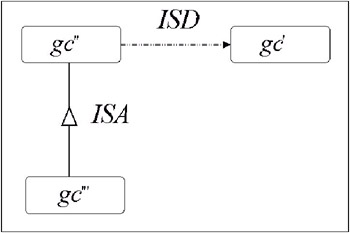Figure 5: The Inheritance of Binding Attribute

Property: The ISD is a partially ordered relation, i.e., it satisfies the following properties.

• reflexivity: Let gc be a geographic class, gc ISD gc.

• antisymmetry: Let gci, gcj be geographic classes where i j. If gci ISD gcj, then gcj ISD gci is not satisfied.

• transitivity: Let gci, gcj, gck be geographic classes where i j and j k. If gci ISD gcj and gcj, ISD gck, then gci ISD gck.

The third property of this relationship indicates that the binding attributes of gck will be included in the set of binding attributes of gci. They are calculated by a roll-up operator composed of a concatenation of complete containment functions along the geographic dimension. Therefore, each covering attribute captures an orthogonal type of inference.

Definition 15: Let C = <Cname, L, fc: LT M> be a cube, and let lg L be a geographic level to which the geographic class gc = λ1(lg) corresponds. The binding attribute of such a class has the schema as follows: AB = <Cname, L lg, fc: L lg}T M>. Let lg L be a geographic level that satisfies lg lg. Then, the Full-Contains relationship between gc = λ1(lg) and gc = λ1(lg) exists. The binding attribute of the geographic class gc by Definition 14 is AB = <Cname, L lg, fc: L lg}T M>. It is obtained from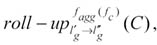, and it is called covering binding attribute.

Example 14: Let the geographic classes PROVINCE and MUNICIPALITY be related by the Full-Contains relationship. As a consequence of this relationship, the binding attribute of the PROVINCE can be inferred from the binding attribute of the MUNICIPALITY (see Figure 6), and each instance of the latter is defined as follows: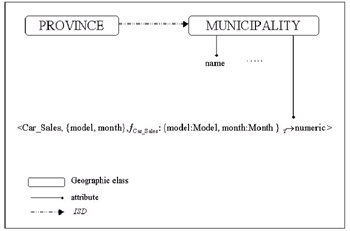Figure 6: Example of Inferring Binding Attribute

For each instance of PROVINCE, for example "Milan," this attribute is calculated by the formula: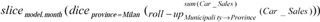A similar line of reasoning can be followed for geographic classes. Indeed, we have the generation of more binding attributes corresponding to different specialized geographic classes that are interrelated by the ISD relationship. This is described by the next steps:

1. the automatic generation of the ISD relationship between each pair of superclasses which are already related by the Full-Contains relationship;

2. for each geographic level lg L of C = <Cname, L, fc: {L}T M >, identify the corresponding geographic class by the function λ in the GDB;

3. if λ1(lg) is a specialization of the geographic class gc, then create the geographic class s gc and make it a sub-class of gc;

4. generate the binding attribute of s gc with the schema AB = <Cname, L lgfc : {L lg}T M >;

5. for each geographic class belonging to the higher level of geographic taxonomy wrt gc, create its specialization;

6. because of Step 1 between any pair of such specialized geographic classes, the ISD relationship exists;

7. the binding attribute of any specialized class s gc, that is next to s gc in the geographic taxonomy, is derived from the s gc's binding attribute through the roll-up operation.

Example 15: We extend the schema of the GDB, which is shown in Figure 1, with the cube illustrated in Example 12. For a clear description, we assume that the production departments are located in municipalities.

` <Shoe_Sales,{Province of distribution, Municiplaity of production, Month},          fShoe_Sales :{Province of distribution: Province, Municiplaity of production : Municipality, month : Month}T → integer > `

Note that in the initial phase of correspondence between the MDDB and the GDB, the classes MUNICIPALITY, PROVINCE, and REGION become superclasses.

Then, we have:

1. the automatic generation of the ISD relationship between each pair of superclasses MUNICIPALITY, PROVINCE, and REGION which are already related by the Full-Contains relationship;

2. the generation of the specialized geographic class PROVINCE OF DISTRIBUTION with the binding attribute

` <Shoe_Sales,{Municiplaity of production, Month},          fShoe_Sales :{Municiplaity of production : Municipality, month : Month}T → integer > `

3. the generation of the specialized geographic class REGION OF DISTRIBUTION and the ISD relationship between this class and the class defined in Step 2;

4. the generation of the geographic class MUNICIPALITY OF PRODUCTION with the binding attribute

` <Shoe_Sales,{Province of distribution, Month},          fShoe_Sales :{Province of distribution : Province, month : Month}T → integer > `

5. the generation of the geographic classes PROVINCE OF PRODUCTION and REGION OF PRODUCTION and ISD relationship between them.

In Figure 7, the result of the above process is shown. Note, each previous labeled step is represented with the same label in the figure.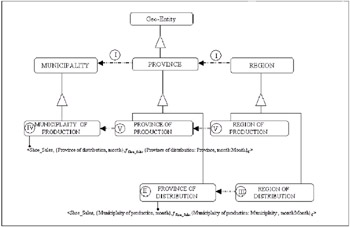Figure 7: Example of Different Steps for the Generation of Specialized Geographic Classes

Analogously in the above discussion, the covering binding attributes are not created in the geographic classes which are at a higher, more aggregate level in the hierarchy.

#### Deduced Attribute

Deduced attributes may result from the aggregation over the local levels of a given basic binding attribute through roll-up and slice operators as follows:

Definition 16: Let C =< Cname, L, fc : LT M> be a cube, and let lg L be a geographic level to which the geographic class gc = λ1(lg) corresponds. Starting from AB = < Cname, L, lg fc : {L lg}T M> the sub-cubes which are obtained by the application of the following operators:

•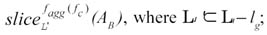•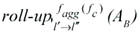where l, l L and they belong to dimension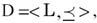, such that l l and fagg is SUM are called deduced binding attributes.

Example 16: From AB = < Car_Sales, {model, month}, fCar_Sales: {model:Model,month:Month}T M>, the deduced binding attributes, by applying slice operator are, for instance: < Car_Sales,{model}, fCar_sales :{model:Model}T M>, < Car_Sales,{month}, fCar_Sales :{month:Month}T M >. Applying the roll-up operator, they are: < Car_Sales, {model, year}, fCar_Sales :{model : Model, year : Year}T M>, < Car_Sales, {vehicle, year}, fCar_Sales :{vehicle: Vehicle, year : Year}T M>.

To compute the deduced binding attributes, we defined a set of methods in the root class Geo-Entity, which are able to invoke a set of OLAP-like operators in the context of geographic classes. They are potentially equivalent to the OLAP operators of MDDBs, and are called G-Slice, G-roll-up, and G-dice. They are inherited by each sub-class of the root class, and each of these operations can be invoked at run time by the substitution of the sub-class parameters. The main functions of such an algorithm is to keep the applications of OLAP operators isomorphic, both to binding attributes in the GDBs and to the cube from which such a binding attribute is calculated in the MDDB. In this way, the processing of a joint query formalized on both databases cannot be perceived by the user.

Depending on each type of operator, the following algorithm shows the schema of resulting cubes or attributes and the relative computation in the context of MDDB through OLAP operators.

#### Number of Binding Attributes

One interesting issue to take into consideration is related to the combinatorial number of cubes which can result from different types of binding attributes. In order to obtain the total number of the basic, covering, and deduced binding attributes, we first give some definitions which will be used in the theorems introduced afterwards.

Let us consider the following symbols: K is the number of different cubes in an MDDB; Ni represents the total number of levels (geographic and non-geographic) of the i-th cube; Gi represents the number of geographic levels of the i-th cube; li,h represents the number of basic and covering binding attributes generated by a geographic level (1 h LG) of the cube; fi,k is the number of binding attributes generated by applying roll-up and slice operators to any local level k 1 of the i-th base cube.

Any geographic level of each independent cube generates a basic binding attribute: in a generic cube Gi geographic levels exist. Therefore, we have the following definition.

Definition 17: Given a set of n cubes. The number of basic binding attributes of each cube i = 1,, n, denoted by Ai(b), is Gi, and the number of basic and covering binding attributes is: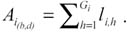We can calculate the total number of the attributes generated by a cube in a geographic class by the next theorem.

Theorem 2: The total number of basic, covering, and deduced binding attributes of the i-th cube is given as follows: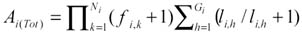Proof: For each base and covering binding attribute, we can apply roll-up and slice operators to the local levels for generating deduced binding attributes. Let Pi,h be the number of local levels of a generic binding attribute and let Qi,h,q, q 1 be the number of deduced binding attributes generated by the application of the roll-up and slice operators to a generic local level. Then, the number of the deduced binding attributes for all local levels is: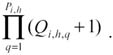From a generic geographic level h, we obtain li,h basic and covering binding attributes; then, the number of deduced binding attributes generated by such a geographic level of the given cube is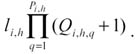Hence, the number of geographic levels is Gi, then the total number of basic, covering, and deduced binding attributes generated by i-th cube is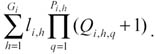As we can observe, the geographic levels of the cube appear twice as li,h and Qi,h,q.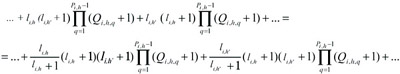where the term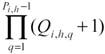refers to non-geographic levels.

Therefore, for all levels Ni we have: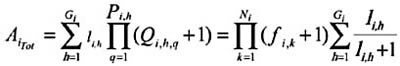Theorem 3: The number of basic binding attributes generated by K independent cubes is: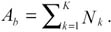The number of base and covering binding attributes generated by K independent cubes is: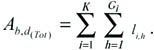Then, the total number of the basic, covering, and deduced functional attributes generated by K cubes is: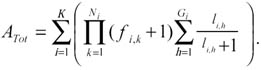Proof: The proof is trivial because the K cubes are independent, then all the results of Theorem 2 are satisfied by them.

To emphasize and clarify the problem of binding attributes blowing up, and to make it easier to understand the general concepts defined in the previous theorems, we give the following examples.

Example 17: Let us consider that an MDDB is composed of the following cubes (K = 3) where facts/measures are recorded for Italy only and the hierarchies are those indicated in Figure 1:

`  < Car_Sales, {city, model}, fCar_Sales : {day : Day, model: Model}T → numeric >  <Shoe_Sales,{Region of production, Region of distribution, Month},          fShoe_Sales :{Region of production : Region, Region of distribution : Region, month : Month}T → numeric > <≠ of patients, {Municipality of residence, Province of hospitalization, Day},          f# of patients :{Municiplaity of residence : Municipality, Province of hospitalization : Province, day : Day}T → numeric > `

The number of base, covering, and deduced binding attributes are indicated in Table 1.

Table 1: Number of Binding Attributes

Basic

Basic + Covering

Basic + Covering + Deduced

Car_Sales

1

3

36

Shoe_Sales

2

2

12

# of patients

2

5

68

All the cubes

5

10

116

This total number has been given against three simple cubes defined by one and two geographic levels between each pair of which there can be a hierarchical or a non-hierarchical relationship. These numbers are not intended to give a comprehensive evaluation of performance. Rather, they demonstrate the other possible answers to queries which can be driven from binding attributes.Multidimensional Databases: Problems and Solutions
ISBN: 1591400538
EAN: 2147483647
Year: 2003
Pages: 150